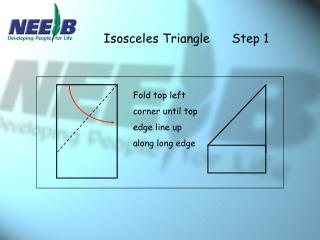# Isosceles Triangle Step 1 - PowerPoint PPT PresentationDownload PresentationIsosceles Triangle Step 1

Isosceles Triangle Step 1
Download Presentation## Isosceles Triangle Step 1

- - - - - - - - - - - - - - - - - - - - - - - - - - - E N D - - - - - - - - - - - - - - - - - - - - - - - - - - -
##### Presentation Transcript

1. Isosceles Triangle Step 1 Fold top left corner until top edge line up along long edge

2. Isosceles Triangle Step 2 Fold left edge of rectangle to fit along the sloping edge.

3. Equilateral Triangle Step 1 Fold in half lengthways and then open out again

4. Equilateral Triangle Step 2 Hold bottom right corner; Fold bottom left corner to centre crease

5. Equilateral Triangle Step 3 Fold the upper part so that the left edge lies along the sloping edge

6. Equilateral Triangle Step 4 Fold small triangle over to line up with edge

7. Kite Step 1

8. Kite Step 2

9. Kite Step 3

10. Kite Step 4

11. Rhombus Step 1

12. Rhombus Step 2

13. Rhombus Step 3

14. Rhombus Step 4

15. Quadrilateral Poster Square Rectangle Rhombus  Kite Parallelogram Trapezium

16. Parallelogram Rectangle Trapezium Square Kite Rhombus

17. 4 equal sides 4 right angles 4 right angles diagonals bisect each diagonals bisect each other at right angles other at right angles diagonals are equal diagonals are equal 4 lines of symmetry opposite sides are parallel opposite sides are parallel opposite sides are parallel

18. rotational symmetry order 4 angles add up to 3600 rotational symmetry order 2 angles add up to 3600 angles add up to 3600 angles add up to 3600 angles add up to 3600 opposite sides are parallel 2 pairs of equal sides opposite sides are equal 2 pairs of equal sides opposite sides are equal and parallel 2 pairs of equal sides